14 January 2018

# Making a building block from GDS

### How to make a building from GDS file

```# example created by Bright Photonics

import nazca as nd

with nd.Cell(name='mmi_bb') as mmi_bb:
filename='mmi_bb.gds',
cellname='mmi'
)
mmi.put()

nd.Pin('a0', width=2).put(0, 0, 180)
nd.Pin('b0', width=2).put(40, 2.5, 0)
nd.Pin('b1', width=2).put(40, -2.5, 0)

bb = mmi_bb.put(0)
# connect to mmi Pin 'b0' and 'b1'
nd.bend(angle=90, width=2, layer=1).put(bb.pin['b0'])
nd.bend(angle=-90, width=2, layer=1).put(bb.pin['b1'])

nd.export_gds()```

In this example we show how to load a MMI GDS building block and add Pins ‘a0’, ‘b0’ and ‘b1’ to the block. Having the Pins set at the input and output ports of the MMI, it is easy to connect to this building block and draw the layout.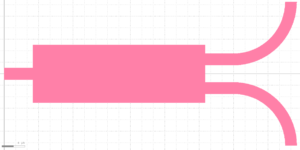We can also add stubs and the boundary around the MMI to better visualize the component.

```# example created by Bright Photonics

import nazca as nd

with nd.Cell(name='mmi_bb') as mmi_bb:
filename='mmi_bb.gds',
cellname='mmi'
)
mmi.put()

nd.Pin('a0', width=2).put(0, 0, 180)
nd.Pin('b0', width=2).put(40, 2.5, 0)
nd.Pin('b1', width=2).put(40, -2.5, 0)

# Put stubs
nd.put_stub()

# Put boundary around the mmi bb
nd.put_boundingbox('org', length=40, width=10)

bb = mmi_bb.put(0)
# connect to mmi Pin 'b0' and 'b1'
nd.bend(angle=90, width=2, layer=1).put(bb.pin['b0'])
nd.bend(angle=-90, width=2, layer=1).put(bb.pin['b1'])

nd.export_gds()```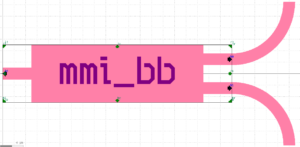## Related Tutorials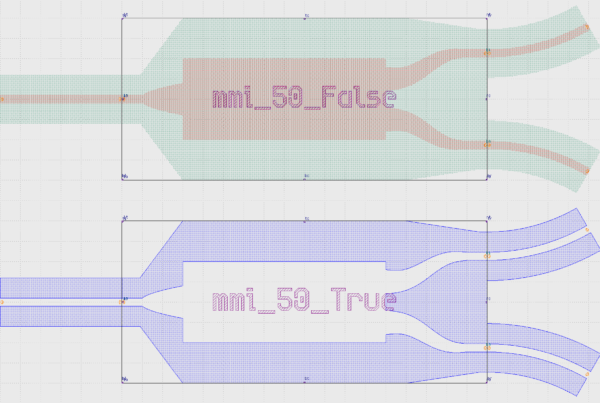In this example we show how to create an inverted MMI with custom interconnects.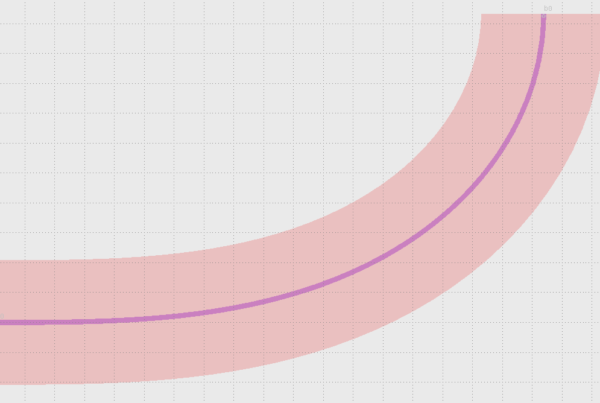In this example we show how to use Euler bends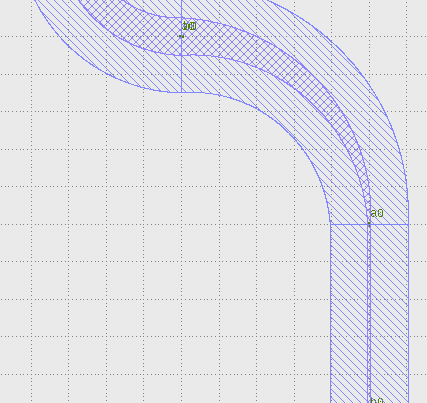In this example we show how to create a free form parametric curve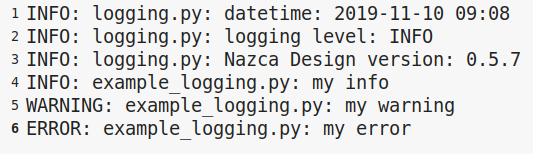In this example we show how to log your layout.